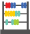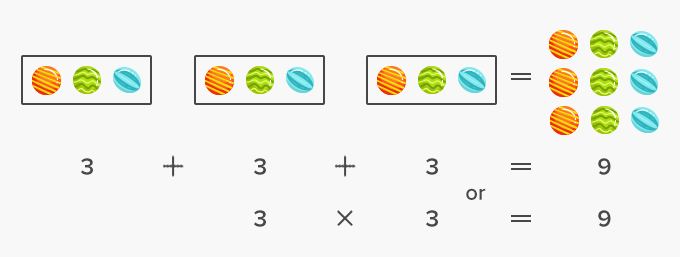# Multiply - Definition with Examples

The Complete K-5 Math Learning Program Built for Your Child

• 40 Million Kids

Loved by kids and parent worldwide

• 50,000 Schools

Trusted by teachers across schools

• Comprehensive Curriculum

Aligned to Common Core

##Let's learn!

What is to multiply?
In math, to multiply means to add equal groups.
When we multiply, the number of things in the group increases.

The two factors and the product are parts of a multiplication problem.

In the multiplication problem, 6 × 9 = 54, the numbers 6 and 9 are the factors, while the number 54 is the product.Here is another example of a multiplication fact that shows multiplication is also repeated addition.Fun Facts The product of any number and zero is always zero. The product of any number and one is the number itself. For every multiplication fact, there are two division facts.

##Let's sing!

One is a factor of all numbers on the shelf,
The number multiplied with it, is the product itself!

##Let's do it!

While solving multiplication word problems, ask your child to try using both the standard algorithm and area model for multiplying numbers.

##Related math vocabulary

Won Numerous Awards & Honors